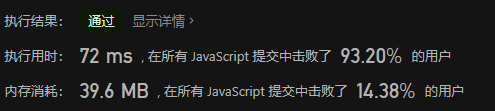# 【LeetCode】最大子序和從O(N^3)到O(N) - 暴力初探 - 分而治之 - 線上處理

theme: fancy highlight: a11y-dark

「這是我參與11月更文挑戰的第29天，活動詳情檢視：2021最後一次更文挑戰

# 53. 最大子序和

https://leetcode-cn.com/problems/maximum-subarray/

## ① 初探：暴力求和

```js /* * @param {number[]} nums * @return {number} / var maxSubArray = function (nums) { let thisSum = -Infinity; let maxSum = -Infinity;

for (let i = 0; i < nums.length; i++) { for (let j = i; j < nums.length; j++) { thisSum = 0; // nums[i] 到 nums[j]的子序和 for (let k = i; k <= j; k++) { thisSum += nums[k]; if (thisSum > maxSum) { // 如果剛得到的這個子列和更大 maxSum = thisSum; // 則更新結果 } } } }

return maxSum; }; ```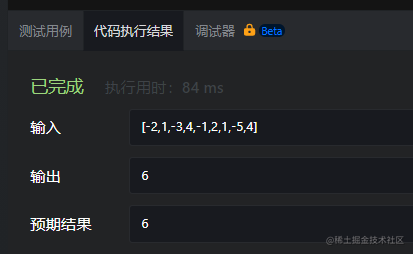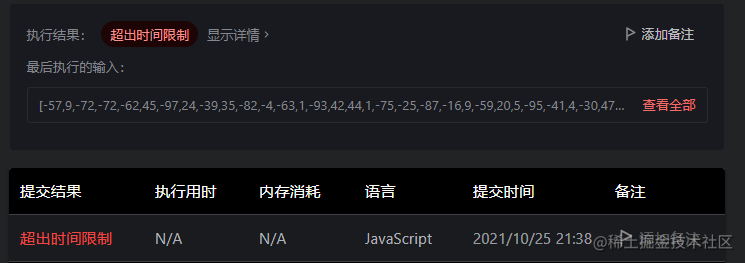## ② 進步：優化求和

for (let i = 0; i < nums.length; i++) { thisSum = 0; // nums[i] 到 nums[j]的子序和 for (let j = i; j < nums.length; j++) { thisSum += nums[j]; // 對於相同的i，不同的j，只要在j-1次迴圈的基礎上累加1項即可 if (thisSum > maxSum) {// 如果剛得到的這個子序和更大 maxSum = thisSum; // 則更新結果 } } }

return maxSum;

}; ```

## ③ 分而治之

1) 把它分成兩個或多個更小的問題；
2) 分別解決每個小問題；
3) 把各小問題的解答組合起來，即可得到原問題的解答。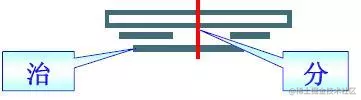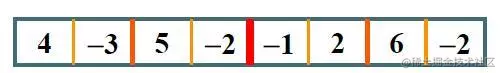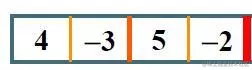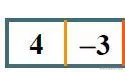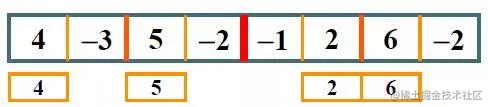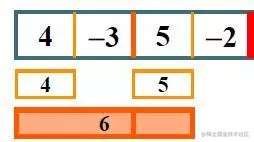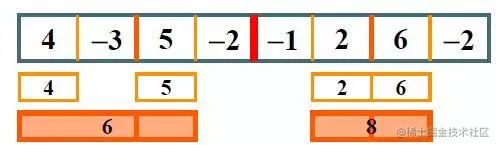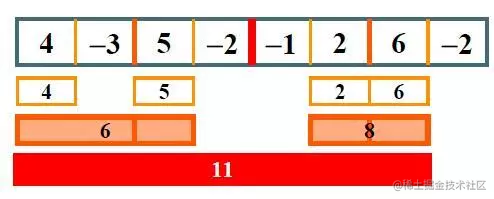```js /* * @param {number[]} nums * @return {number} / function maxSubArray(nums) { let len = nums.length

``````if(len === 0){
return 0
}

return maxSubArraySum(nums, 0, len - 1)
``````

};

// 計算交叉的最大子序和 function maxCrossingSum(nums, left, mid, right){ let sum = 0 let leftSum = -Infinity for(let i = mid; i >= left; i--){ sum += nums[i] if(sum > leftSum){ leftSum = sum } }

``````sum = 0
let rightSum = -Infinity
for(let i = mid + 1; i <= right; i++){
sum += nums[i]
if(sum > rightSum){
rightSum = sum
}
}
return (leftSum + rightSum)
``````

}

// 計算最大子序和函式【遞迴】 function maxSubArraySum(nums, left, right){

``````if(left === right){
return nums[left]
}

let mid = Math.floor((left + right)/2)

let leftSum = maxSubArraySum(nums, left, mid)
let rightSum = maxSubArraySum(nums, mid+1, right)
let crossSum = maxCrossingSum(nums, left, mid, right)

return max3(leftSum, rightSum, crossSum)
``````

}

// 返回3個數中的最大值 function max3(num1, num2, num3){ return Math.max(num1, Math.max(num2, num3)) } ```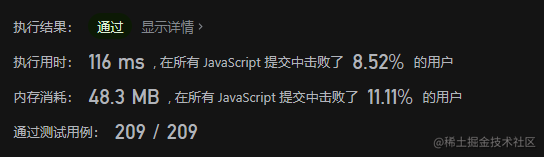## ④ 分治優化：線段樹

```js function Status(lSum, rSum, mSum, iSum) { this.lSum = lSum; // lSum 表示 [l,r] 內以 l 為左端點的最大子段和 this.rSum = rSum; // rSum 表示 [l,r] 內以 r 為右端點的最大子段和 this.mSum = mSum; // mSum 表示 [l,r] 內的最大子段和 this.iSum = iSum; // iSum 表示 [l,r] 的區間和 }

function pushUp(lSub, rSub){

``````// iSum 就是 左子區間和 + 右子區間的和
const iSum = lSub.iSum + rSub.iSum;

// lSum 就是 左子區間的lSum 和 左區間
const lSum = Math.max(lSub.lSum, lSub.iSum + rSub.lSum);

// rSum 表示 [l,r] 內以 r 為右端點的最大子段和
const rSum = Math.max(rSub.rSum, rSub.iSum + lSub.rSum);

// mSum 表示 [l,r] 內的最大子段和
const mSum = Math.max(Math.max(lSub.mSum, rSub.mSum), lSub.rSum + rSub.lSum);

return new Status(lSum, rSum, mSum, iSum);
``````

}

// 查詢 a 序列 [l,r] 區間內的最大子段和 function getInfo(a, l, r){ // 遞迴終止條件 if (l === r) { return new Status(a[l], a[l], a[l], a[l]); }

``````// 下面是"分"的過程
// 得到中間值
const m = (l + r) >> 1;
// 分治：求左邊
const lSub = getInfo(a, l, m);
// 分治：求右邊
const rSub = getInfo(a, m + 1, r);

return pushUp(lSub, rSub);
``````

}

var maxSubArray = function(nums) { return getInfo(nums, 0, nums.length - 1).mSum; }; ```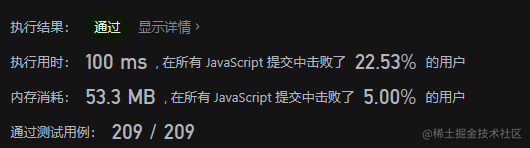## ⑤ 線上處理

`thisSum` 維護一個 向右累加 子序和 如果之前和子序和都沒有第`i`個元素大，就從`i`開始重新維護一個 累加子序和

`maxSum` 儲存遍歷過程中的最大子序和

```javascript /* * @param {number[]} nums * @return {number} / var maxSubArray = function(nums) { // 當前子序和 let thisSum = 0; // 最大子序和 let maxSum = nums;

``````// 遍歷一遍序列
for(let i = 0; i < nums.length; i++){

if(thisSum + nums[i] < nums[i]){
thisSum = nums[i];
}else{
thisSum += nums[i];
}

if(thisSum > maxSum){
maxSum = thisSum;
}
}

return maxSum;
``````

}; ```

```javascript /* * @param {number[]} nums * @return {number} / var maxSubArray = function(nums) { let thisSum = 0; let maxSum = nums;

``````for(let i = 0; i< nums.length; i++){
thisSum = Math.max(thisSum + nums[i], nums[i]);
maxSum = Math.max(maxSum, thisSum);
}

return maxSum;
``````

}; ``` 這裡的時間複雜度是\$\$O(N)\$\$， 空間複雜度是\$\$O(1)\$\$